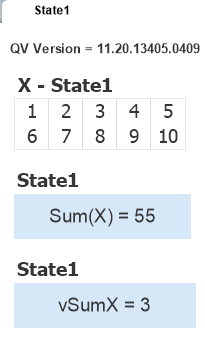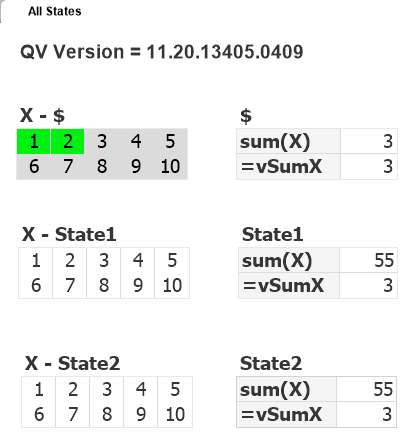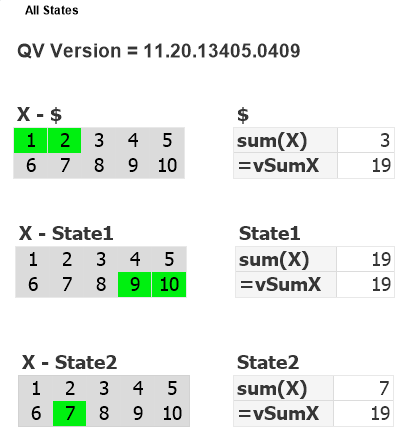QV12 Variables with Alt State Fixed

Summary:  QV11 contained an inconsistency in how variables with equal signs were evaluated when using Alternate States.  This has been fixed in QV12.10.  Read on if you want the details.

QlikView V12.10 includes an important fix to variable evaluation when using alternate states. A quote from the Release Notes:

In QV11.20 the variable was expanded in the first state encountered and this resulted in a random behavior when more than one alternate state was being used. Whereas in version 12 and up, the variable always belongs to a specific alternate state and this results in different behavior.

The random behavior described in QV11.20 has generated several interesting posts to QlikCommunity with responses of “I can reproduce” / “I can’t reproduce” and few clear answers.

I find the problem confusing and interesting enough to warrant an explanation and example beyond the Release Note.

What I am describing in this post only affects variables with a leading “=” in the definition, e.g. “=Sum(Sales)”.  These variables are calculated once in the context of the entire document. They are not calculated per row in a chart.

Let’s consider a variable named “vSumX” with a definition of “=Sum(X)”.  The expression simply sums all selected values of X.   Suppose we have two States in our document — “Default” and “State1”.  There could be two different selections for “X”.  Which set of X should the variable sum?

If we consider the variable definition in isolation, the answer is “Default set”  as there is no set identifier in the expression.  But what if the variable is referenced in an object in State1.  Should the State1 values of X be used?

No matter what you think the rules should be, here’s what was happening in QV11.20.  The variable was expanded (evaluated) in the first state encountered.  First state encountered means first state in the calculation chain, not something the developer directly controls.

Let’s look at some examples.   I’ve created a sample app (you can download here) with three States — Default,  State1 and State2.  The variable “vSumX” is defined as “=Sum(X)”.

With all objects on sheet in the Default state, selections in X would yield results like this.  (Note “\$” indicates default state).The first text box contains the expression “Sum(X)”.  The second text object contains the reference to variable vSumX.  The two values are what we might expect, summing the selected values of X in this state.

Let’s switch to a sheet that contains objects in the state named “State1”.No selections in X and the first text object shows the expected result.  The second object shows the value of vSumX as previously calculated from the default state.  If we make selections on this State1 sheet, that will cause vSumX to be recalculated and both State1 and the Default sheet will reflect the State 1 number.  Is that correct?  Is it useful?  It’s at least consistent and comprehensible.

My next example is where the aforementioned “random” comes into play.  Let’s put objects from three states on the same sheet.I’ve selected some values in the Default state of X and the results are what I might expect.  The value of vSumX is calculated from my last selections and the variable value is consistent across objects — there is only ever one value for a variable at a given point in time.Now I select some X values in State1 and expect to see a new value (19) for vSumX.  But no change! The variable was expanded (evaluated) in the first state encountered which happened to be Default (\$).Now I select some X values in State2.  If the vSumX calc used my last selection I would expect to see 7.  But no, I see 19.  The State1 values were used.  If I repeat the exercise, it may use a different state to calc vSumX.  If you test you may get different results.  In this last example, State1 was used because it was the first state encountered in the calculation chain.  The order is not consistent.  It will be influenced by factors such as number of available processors and the order in which the objects were created.

Now that we’ve established that QV11,20 is broken in this regard, how was it fixed in QV12.10?  Simple.  QV12 uses  set identifiers as specified in the expression, without inheritance.

=Sum(X)

will use the Default State as there is no identifier.   If you want to Sum from a specific state, use it in the expression:

=Sum({State1} X)

Variables do not belong to any State.  Aggregation functions used in a variable may specify a Statename, just as chart expression do.  The difference is that the absence of a set identifier in a chart expression means “inherit the state from the containing object”.  In a “=” variable, no set identifier means “use the default state”.

A reminder that end of standard support for QV11 comes on Dec 8, 2017.  If you haven’t yet upgraded to QV12.10, I encourage you to do so.  Download my  QV12 Upgrade Considerations Doc as part of your planning process.  Feel free to contact me if you want some assistance with your upgrade.

-Rob

Update:  Qlik has extended support for QV11.20 to March 31, 2018.

10 thoughts on “QV12 Variables with Alt State Fixed”

1.Bill Lay says:

Great post Rob.

I see the v.12.10 “fix” as eroding the value of alternate states somewhat, in the sense that one can’t simply reuse the same variable in different states and get the selection space of that state. (If I’m understanding your analysis.)
Is it possible to pass a statename into a variable? That could be the workaround:
SET vSum = ‘=sum({\$1} X)’;

called as: \$(=vSum(State1))

or ??

1.Rob Wunderlich says:

I understand what your are thinking, but….The most common use case of a re-usable variable in multiple states would be an expression like “Sum(Sales)” (without the equal sign). That would evaluated in the state of the chart, so doesn’t apply here.

A variable defined with a leading equal sign:
SET vSum = ‘=sum({\$1} X)’;
cannot contain parameters as it is evaluated every time data changes, not when called.

1.Diricx Timmy says:

Hi, i tried to use this solution for a customer who are using the alternate states with variables in combination with an aggr() function.
Example:
=> Sum(Aggr(Sum(1),\$(vAggrLevel_Evo)))
They use a different alternate state on the variable vAggrLevel_Evo which is used in an aggr() function to have a dynamic aggregation functionality.

Before (in version 11.2) they used a “=” in the variable. I deleted this in the 12.1 tests and in a simple textbox (with the alternate state changed), the variable gives the correct result. But in the aggregation function not (because it doesn’t return a result)

Is the use of a variable with alternate states IN an aggr() function also changed in version 12.1?

1.Rob Wunderlich says:

I don’t think you are seeing a release change, but rather the results of removing the “=” from the variable def. I’m assuming vAggrLevel_Evo is an expression that returns a string fieldname. Add the “=’ into the chart expression like this:

Sum(Aggr(Sum(1),\$(=vAggrLevel_Evo)))

1.Timmy says:

Hi Rob,
My issue got solved. I will give a short resume of what the problem was and what the solution is:
I was using in the 11.2 version an aggregation function Sum(Aggr(Sum(1),\$(vAggrLevel_Evo))) in to different alternate state (default state and manually created alt. state). In 11.2, there was apparently a bug that could cause some weird results in relation with using variables and alternate states (see some earlier post on qlikviewcookbook.com for more details).
In the variable vAggrLevel_Evo, we used an expression/code as in this example:
=If(checkfield=1,result1,result2)

The issue is that we used an “=” sign in the expression and this is causing that the expression is always evaluated in the default state. So I thought, then we used the “=” sign and everything is solved but noooo 😉
We also needed to change the way we used our variable in the aggr() function.

Solution:
Delete the “=” sign in the variable expression and use this kind of variable evaluation in an aggr() function:
Sum(Aggr(Sum(1),\$(=\$(vAggrLevel_Evo))))
This “\$(=)” in this variable declaration will cause the expression in the variable to be evaluated and also taking into account in which alternate state the chart/textbox/… is currently stated “\$(=\$(var))”

2.Eerno Niemenmaa says:

Thanks for yet another great post Rob!

It seems you can achieve this functionality by defining the variable (vSum) like this:
\$(=sum({\$1}X))
And calling it like this (for example in a chart, “State1” being the name of the alt state):
=\$(vSum(State1))

3.Marcel Groothuis says:

Hi Rob,

In my case the expression for my variable is =If(GetSelectedCount([field a])=0 and GetSelectedCount([field b)=0,1,0)

I can’t get it to work on a sheet that has an alternate state: the content will always be 0 ;(

Regards,

Marcel

1.Rob Wunderlich says:

Marcel,
You will need to specify the statenameas the 3rd parm in the function like:

=If(GetSelectedCount([field a], False(), ‘mystate’)=0 and GetSelectedCount([field b], False(), ‘mystate’)=0,1,0)

2.Michael says:

Hello Rob,

I am having a problem using variables in version 12 of Qlikview Desktop and Qlikview Server, version 11.
With version 11 of Qlikview Desktop it was working.

I have two Set Variable actions, OnOpen and OnPostReload, which loads a variable with the result of another variable.

Variable
vMyVariable

Value
= vMyValue

vMyVariable value is a string.

Being logged in as a user, through the internet browser, I can see that after the document reload / update, the variable is not being set, or worse, it is clearing its value.

Only works if I take the = sign, so:.

Variable: vMyVariable
Value: vMyValue

Can you tell me something about it?

* There are no alternative states

1.Rob Wunderlich says:

Sorry for the delayed response. I suggest taking this question to the QlikCommunity (if you haven’t already) where it will be easier to look at some sample data and follow up questions.